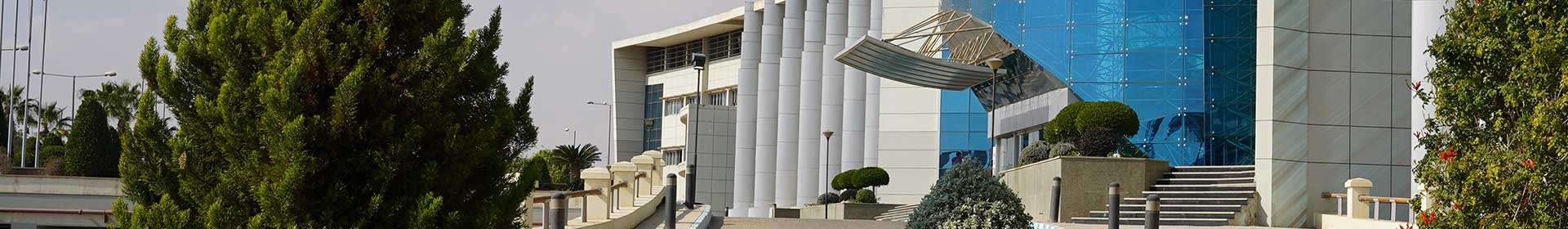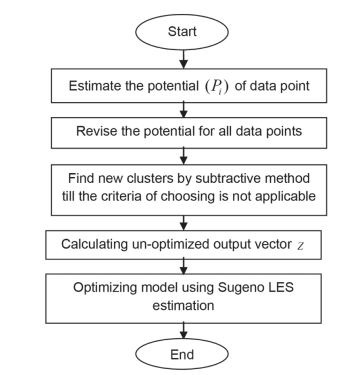Journal

# Predicting telecommunication tower costs using fuzzy subtractive clustering

By
Marzouk M.
Alaraby M.

This paper presents a fuzzy subtractive modelling technique to predict the weight of telecommunication towers which is used to estimate their respective costs. This is implemented through the utilization of data from previously installed telecommunication towers considering four input parameters: a) tower height; b) allowed tilt or deflection; c) antenna subjected area loading; and d) wind load. Telecommunication towers are classified according to designated code (TIA-222-F and TIA-222-G standards) and structures type (Self-Supporting Tower (SST) and Roof Top (RT)). As such, four fuzzy subtractive models are developed to represent the four classes. To build the fuzzy models, 90% of data are utilized and fed to Matlab software as training data. The remaining 10% of the data are utilized to test model performance. Sugeno-Type first order is used to optimize model performance in predicting tower weights. Errors are estimated using Mean Absolute Percentage Error (MAPE) and Root Mean Square Error (RMSE) for both training and testing data sets. Sensitivity analysis is carried to validate the model and observe the effect of clusters radius on models performance. Copyright © 2015 Vilnius Gediminas Technical University (VGTU) Press.